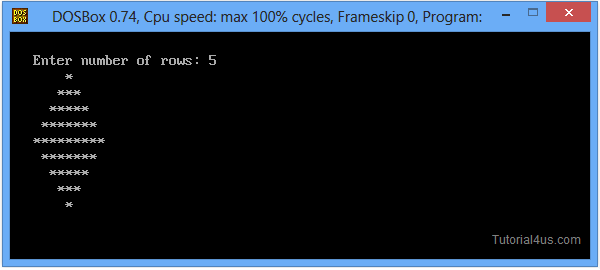# C++ Program to Print Diamond Pattern

## C++ Program to Print Diamond of Stars

Using C++ language you can print diamond of stars, here you need to print two triangle, simply print first triangle and second triangle is reverse of first triangle.

## C++ Program to Print Diamond of Stars

```
#include<iostream.h>
#include<conio.h>

void main()
{
int n, c, k, space = 1;
clrscr();
cout<<"\n\nEnter number of rows: ";
cin>>n;
space = n - 1;

for (k = 1; k<=n; k++)
{
for (c = 1; c<=space; c++)
cout<<" ";

space--;

for (c = 1; c<= 2*k-1; c++)
cout<<"*";

cout<<"\n";
}

space = 1;

for (k = 1; k<= n - 1; k++)
{
for (c = 1; c<= space; c++)
cout<<" ";

space++;

for (c = 1 ; c<= 2*(n-k)-1; c++)
cout<<"*";

cout<<"\n";
}
getch();
}
```

Output## Pure VPN Privide Lowest Price VPN Just @ \$1.65. Per Month with Non Detected IP Lowest Price Non Detected IP VPN